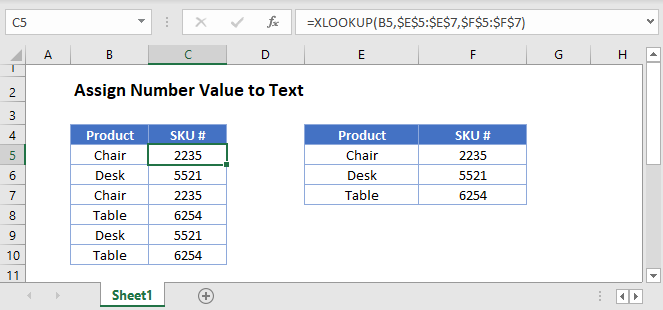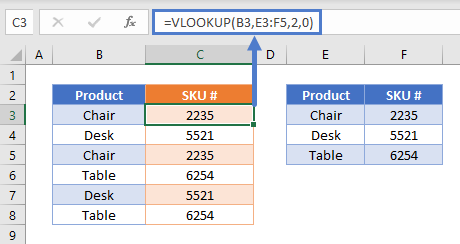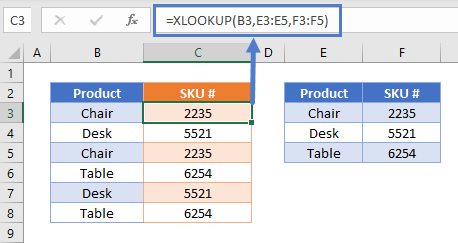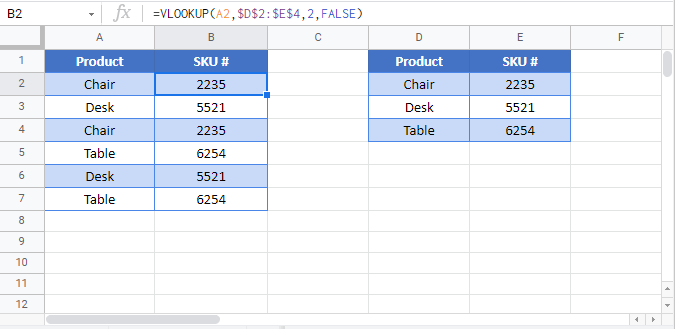# Assign Number Value to Text – Excel & Google Sheets

This tutorial will demonstrate how to assign a numerical value to a text string.## VLOOKUP Method

To assign a number value to a text string, we can use the VLOOKUP Function along with our lookup table. In this example, we assign a SKU number to each product like so:

``=VLOOKUP(B3,E3:F5,2,0)``This formula finds “Chair” in the range E3:F5 and returns the second column in that row: “2235”. (The “0” argument specifies that we need an exact match.)

## XLOOKUP Method

Another method is to use the XLOOKUP Function to achieve the same results:

``=XLOOKUP(B3,E3:E5,F3:F5)``Instead of a range and column index number, the XLOOKUP Function takes a lookup array and a return array.

## Locking Cell References

To make our formulas easier to read, we’ve shown the formulas without locked cell references:

``=VLOOKUP(B3,E3:F5,2,0)``
``=XLOOKUP(B3,E3:E5,F3:F5)``

But these formulas will not work properly when copy and pasted elsewhere within your Excel file. Instead, you should use locked cell references like this:

``=VLOOKUP(B3,\$E\$3:\$F\$5,2,0)``
``=XLOOKUP(B3,\$E\$3:\$E\$5,\$F\$3:\$F\$5)``